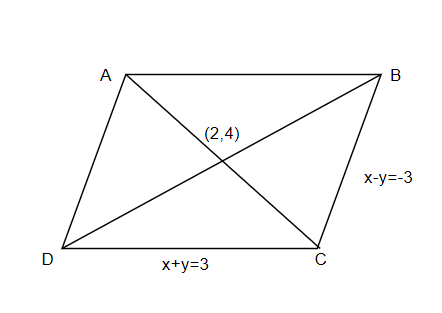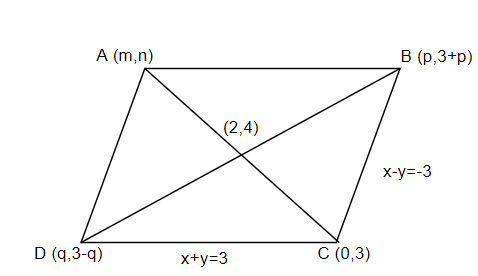Courses
Courses for Kids
Free study material
Free LIVE classes
MoreLIVE
Join Vedantu’s FREE Mastercalss

# Two sides of a parallelogram are along the lines $x+y=3$ and $x-y+3=0$. If its diagonalsintersect at $(2,4)$, then one of its vertex is(a) $(2,6)$(b) $(2,1)$(c) $(3,5)$(d) $(3,6)$Verified
366.3k+ views
Hint: Solve the 2 line equations to find the coordinates of intersection of these 2 lines and use section formula to find the remaining coordinates of a parallelogram.

The equations given in the question are,
$x+y=3$ and $x-y+3=0$
They can be rewritten as,
$x+y=3$ and $x-y=-3$
Also, it is given that the diagonals intersect at $(2,4)$. Draw the parallelogram representing the given data as shown below,AThe vertex C is a point that intersects the lines DC and BC. Therefore, the coordinates of the C can be obtained by adding and solving the two equations of the lines as below,
\dfrac{\begin{align} & x+y=3 \\ & x-y=-3 \\ \end{align}}{\begin{align} & 2x=0 \\ & x=0 \\ \end{align}}
Substituting the value of $x$, we have the value of $y$ as,
\begin{align} & 0+y=3 \\ & \Rightarrow y=3 \\ \end{align}
Therefore, the coordinates of C are $(0,3)$.
The coordinates of vertex B can be obtained by supposing $x=p$in the equation $x-y=-3$. So, we have the coordinates as $(p,3+p)$. To get the coordinates of vertex D, suppose $x=q$in the equation $x+y=3$. So, we have the coordinates as $(q,3-q)$. Let the coordinates of vertex A be $(m,n)$. The figure can be redrawn with the above details as below,The coordinates of the point of intersection of the diagonals are given as $(2,4)$. The point of intersection is the midpoint of the line AC as well as the line BD. It divides the lines in the ratio $1:1$.
Using the section formula, the coordinates of vertex A $(m,n)$ can be found as,
$\left( \dfrac{m+0}{2},\dfrac{n+3}{2} \right)=\left( 2,4 \right)$
Equating both coordinates on either sides,
\begin{align} & \dfrac{m}{2}=2,\dfrac{n+3}{2}=4 \\ & m=4,n=(8-3) \\ & m=4,n=5 \\ \end{align}
Therefore, coordinates of A are $(4,5)$.
Using section formula to find the coordinates of D and B,
\begin{align} & \left( \dfrac{p+q}{2},\dfrac{3+p+3-q}{2} \right)=(2,4) \\ & \left( \dfrac{p+q}{2},\dfrac{p-q+6}{2} \right)=(2,4) \\ \end{align}
Equating both coordinates on either sides,
\begin{align} & p+q=4,p-q=(8-6) \\ & p+q=4,p-q=2 \\ \end{align}
We get two equations $p+q=4$ and $p-q=2$. Adding and solving the two equations,
\dfrac{\begin{align} & p+q=4 \\ & p-q=2 \\ \end{align}}{\begin{align} & 2p=6 \\ & p=3 \\ \end{align}}
Substituting the value of $p$, we have the value of $q$ as,
\begin{align} & 3+q=4 \\ & q=1 \\ \end{align}
Now, re-substituting these values, we can get the coordinates of B and D as,
B$(p,3+p)\Rightarrow (3,3+3)=(3,6)$
D$(q,3-q)\Rightarrow (1,3-1)=(1,2)$
Looking at the options, the only possible answer is the coordinates of B, which is $(3,6)$.
Therefore, we get option (d) as the correct answer.
Note: One way in which this problem could go wrong is by interchanging the coordinates of B and D which are written in terms of $p$ and $q$. If you write the coordinates as $(3+p,p)$ and $(3-q,q)$ instead of $(p,3+p)$and $(q,3-q)$, you might end up getting the coordinates as $(6,3)$ and $(2,1)$ and choose the wrong option.
Last updated date: 25th Sep 2023
Total views: 366.3k
Views today: 3.66k